MainTutorialsOrganic ChemistryPractice TestsOnline QuizzesReference ToolsNuclear Chemistry By Hao ZhangCharges of Elemental Components Of the three elemental components, a proton has a positive charge, a neutron has a neutral charge, and the electron has a negative charge. Atomic Definitions The atomic number (designated by the symbol Z) represents the number of protons in the nucleus. In an element, which never has a charge, the number of protons equals the number of electrons. The mass number (A) is the sum of neutrons and protons in the nucleus. Therefore, A - Z equals the number of neutrons in a nucleus.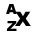Typical Nuclide(where X equals any element): Two Types of Atomic Stability Kinetic stability- the likelihood of a nucleus to change into a more stable state. Thermodynamic stability- A comparison of the potential energy of the nucleus to the sum of the potential energy of its protons and neutrons. A nuclide becomes more stable through radioactive decay. Alpha particle (a ) decay-emission of helium particle: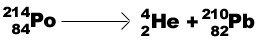Beta particle (b -)decay- emission of an electron: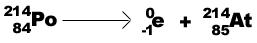Positron (b +) emission-a positively charged electron: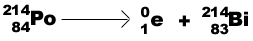Electron capture (b -)-a gaining of electrons:When a positron and an electron are combined, the result is 2 gamma rays. This process is called annihilation.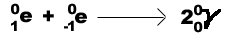Gamma ray (g )-high energy photons that accompany nuclear decays and particle reactions. Since they have neither mass nor charge, they are usually disregarded in equations.*Gamma rays are only produced in specific reactions. Some nuclides exhibit a "zone of stability" where radioactive decay does not take place. Light nuclides with proton/neutron ratio of 1 are stable. Even number of protons and neutrons are more stable than odd numbered. Special number of protons and neutrons are more stable. The "magic numbers" are the following: 2, 8, 10, 28, 50, 82, and 126. All nuclides with Z = 84 are unstable. Rates of Decay All radioactive decay reactions are 1st order. For a 1st order reaction: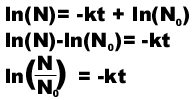The half-life equation for a nuclear reactionNuclear transformation is the conversion of one element to another. Two methods of nuclear transformation: 1. Cyclotron - A particle is accelerated through a particle accelerator and is penetrated into the nucleus. 2. Linear Accelerator - particles accelerated by magnetic fields bombards the nucleus. Fission isthe splitting of a heavy nuclide into two smaller nuclides. Excess neutrons and energy is produced in the reaction. For a chain reaction, the emitted neutrons split nearby nuclides that produce a self-sustaining reaction. Types of Fission reactions Subcritical: less than one neutron splits one nuclide  (This reaction will not be self-sustaining.In order to be self-sustaining, at least one neutron must go on to split another nuclide) Critical: one neutron splits one nuclide Supercritical: more than one neutrons splits one nuclide Fusion-combining two smaller nuclides to form one heavy nuclide. Effects of Radiation During radioactive decay, the nuclides emit radiation. If the nuclide has a large half-life, the decomposition process is very slow. If a nuclide enter into a human body, it will remain there for a long period of time. If the nuclide has a short half-life, the rapid emission of radiation causes a shorter period of greater damage. Rads (radiation absorbed dose) -measurement of radiation 1 rad=10-2J / kg tissue Rem (roentgen equivalent for men)-measures both dosage of radiation and its effectiveness in the environment. 1 rem=#of rads * RBE RBE-the effectiveness of radiation to cause biological damage.news | about us | contact us tutorials index | organic chemistry | practice tests | online quizzes | reference tools site copyright (c) 2002-2013 Learn ChemSubscribe to our low volume newsletter to receive up-to-date information about the CHEM SITE